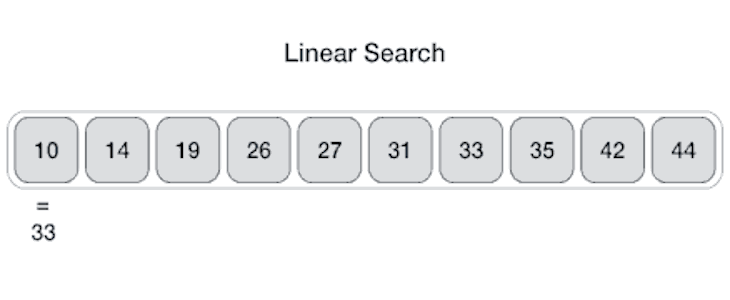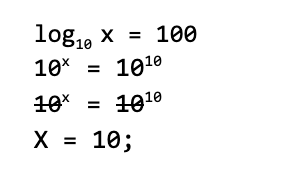# Understanding linear and binary search in JavaScriptIn this tutorial, I’ll begin by explaining a few terms that’ll help us understand this concept.

So, to start off: an algorithm is a set of instructions given to a computer to perform a particular task.

Depending on the task you need to perform, an algorithm will perform it faster or more efficiently. Engineers look at this trade off when creating an algorithm for a given task.

We’ll be looking at how this plays out as we discuss linear (simple) search vs binary search.

## Linear search

Sometimes called simple search, linear search is a method for finding an element within a list.

Suppose we have a list of numbers — let’s say, from 1 to 1000 — and we’re looking for a number in between these parameters. With simple search, we have look through each number one after the other til we find our match.

This means that — worst case scenario — we would have to look through the entire list before we can be sure of a match or be sure we don’t have a match.Check out the JavaScript implementation of linear search below:

```const linearSearch = (list, target) => {
for (let i = 0; i < list.length; i++){
if( list[i] === target) return i
}
return null;
}

const list = [1,2,3,4,5,6,7,8,9,10]
let result = linearSearch(list, 8);
console.log(result); // 8
result = linearSearch(list, 19);
console.log(result); // null```

## Binary search

Binary search, on the other hand, is a better way to search.

Suppose we are looking for the meaning of the word `Organic` in the dictionary.

We would open to the middle and start searching from there rather than starting from the first word that starts with `A`. We can do this because we know that the words in the dictionary are arranged in alphabetical order `(sorted)`, and when we start at the middle we eliminate the need to search through a particular half of the dictionary.

This is synonymous with how we can think of binary search.

It takes in a sorted list and searches for a target. If the target exists, it returns it. If it doesn’t, it returns null.

Because it is a sorted list, we can assume a few things and come up with a pseudocode as follows:

• Start from the value in the `middle` of the list and compare this with the target value
• If the target is equivalent to the value of `middle`, return middle
•  If the target is less than the value of `middle`, recalculate `middle` such that it is increased
• If the target is greater than the value of `middle`, recalculate `middle` such that it is decreased
• Continue this while there is still an item to search for, or return `null`

Let’s look at this diagrammatically with the JavaScript implementation of binary search:

```const binarySearch = (list, target) => {
let low = 0;
let high = list.length - 1;
let guess, mid;

while (low <= high) {
mid = Math.floor((low + high) / 2);
guess = list[mid];
if (guess === target) return mid;
if (guess < target) low = mid + 1
else high = mid - 1;
}
return null;
}```

Essentially, for every guess we make when using binary search we eliminate half of the list.

Let’s assume we have a list of 240,000 numbers and we want to search for a particular number. At most, we would have to go through 18 steps:

```240K
→ 120k ------- 1
→ 60k -------- 2
→ 30 ---------- 3
→ 15k --------- 4
→ 7.5k -------- 5
→ 3.75k ------- 6
→ 1875 --------- 7
→ 938  --------- 8
→ 469 ---------- 9
→ 235 ---------- 10
→ 118 ---------- 11
→ 59 ----------- 12
→ 30 ----------- 13
→ 15 ----------- 14
→ 8 ------------ 15
→ 4 ------------16
→ 2 ------------17
→ 1. ------- 18```

For a simple search, we would be required to go through every number on the list.

## Big O notation

Big O notation is a way we describe how fast or how complex an algorithms is.

When we are adopting an algorithm for a particular problem, we often use this as a tool to understand the trade offs that are available.

It gets its name from the O place before the number of operations that is typically specified in logarithms.

Logarithms can be thought of as exponents — ie, how many of a number you multiply to get another number, etc.### Simple search

Let’s assume we have `n` items on a list. Simple search needs to go through every item on that list, hence we have `n` operations. As a result, the running time in big O notation is O(n);

### Binary search

The big O notation for binary search is O(log n). This is in base two, which is because for every operation we divide the list into two.

## Conclusion

The algorithms that we decide to employ can either improve or hamper the performance of our application hence it is important to properly consider the tradeoff from time to time when adopting a certain algorithm.

You can delve deeper into linear and binary search here.

## LogRocket: Debug JavaScript errors more easily by understanding the context

Debugging code is always a tedious task. But the more you understand your errors the easier it is to fix them.

LogRocket allows you to understand these errors in new and unique ways. Our frontend monitoring solution tracks user engagement with your JavaScript frontends to give you the ability to find out exactly what the user did that led to an error.

LogRocket records console logs, page load times, stacktraces, slow network requests/responses with headers + bodies, browser metadata, and custom logs. Understanding the impact of your JavaScript code will never be easier!

.